RS Aggarwal Class 10 Solutions Chapter 18 - Areas Of Circle Sector And Segment Ex 18A (18.1)

RS Aggarwal Class 10 Chapter 18 - Areas Of Circle Sector And Segment Ex 18A (18.1) Solutions Free PDF

Question 1: The perimeter of a certain sector of a circle of radius 6.5 cm is 31 cm. Find the area of the sector.

Solution:

Given: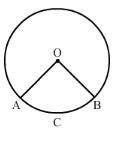Let O be the centre of the circle with radius 6.5 cm and OACBO be its sector with perimeter 31 cm.

Thus, we have:

OA + OB + arc AB = 31 cm

$\Rightarrow$ 6.5 + 6.5 + arc AB = 31

$\Rightarrow$ arc AB = 31 – 13

$\Rightarrow$ arc AB = 18 cm

Now,

Area of the sector OACBO = $\frac{1}{2}\times Radius\times Arc$ = $\frac{1}{2}$ x 6.5 x 18 = 58.5 cm2

Question 2: The radius of a circle is 17.5 cm. Find the area of the sector enclosed by two radii and an arc 44 cm in length.

Solution:

Given:

Length of the arc = 44 cm

Now,

Length of the arc = $\frac{2\Pi r\Theta }{360}$

$\Rightarrow$ 44 = $2\times \frac{22}{7}\times 17.5\times \frac{\Theta }{360}$

$\Rightarrow$ $\Theta =\frac{44\times 7\times 360}{44\times 17.5}$

$\Rightarrow$ $\Theta$ = 144o

Also,

Area of the sector = $\frac{\Pi r^{2}\Theta }{360}$ = $\frac{22}{7}\times 17.5\times 17.5\times 144$ = 385 cm2

Question 3: Two circular pieces of equal radii and maximum area, touching each other are cut out from a rectangular cardboard of dimensions 14 cm x 7 cm. Find the area of the remaining cardboard.

Solution:

We know that we can cut two circular pieces of equal radii and maximum area from the rectangular cardboard whose diameter is equal to the width of the rectangular cardboard.

Therefore, Radii of two circular pieces = Half of the width of the rectangular cardboard = 3.5 cm

Now,

Area of remaining cardboard = Area of rectangular cardboard – 2 x Area of circular piece having radius 3.5 cm

= 14 x 7 – 2($\frac{22}{7}$ x 3.5 x 3.5) = 98 – 77 = 21 cm2

Hence, the area of the remaining cardboard is 21 cm2

Question 4: In the given figure, ABCD is a square of side 4 cm. A quadrant of a circle of radius 1 cm is drawn at each vertex of the square and a circle of diameter 2 cm is also drawn. Find the area of the shaded region. [Use $\Pi$ = 3.14]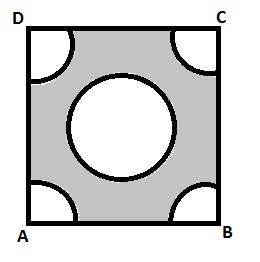Solution:

Area of the square ABCD = (Side)2 = 42 = 16 cm2

Area of the circle = $\Pi r^{2}$

Area = 3.14 x (1)2 = 3.14 cm2

Area of the quadrant of one circle = $\frac{1}{4}\Pi r^{2}$ = $\frac{1}{4}\times 3.14\times 1^{2}$ = 0.785 cm2

Area of the quadrants of four circles = 0.785 x 4 = 3.14 cm2

Area of the shaded region = Area of the square – Area of the circle – Area of the quadrants of four circles = 16 – 3.14 – 3.14 = 9.72 cm2

Question 5: From a rectangular sheet of paper ABCD with AB = 40 cm and AD = 28 cm, a semi-circular portion with BC as diameter is cut off. Find the area of the remaining paper.

Solution:

We know that the opposite sides of triangle are equal

Therefore, AD = BC = 28 cm

Now, Radius of semicircle portion = $\frac{1}{2}$ BC = 14 cm

Therefore, Area of remaining paper = Area of rectangular sheet – Area of semicircular portion

= $40\times 28-\frac{1}{2}\left ( \frac{22}{7}\times 14\times 14 \right )$ = 1120 – 308 = 812 cm2

Hence, the area of the remaining paper is 812 cm2

Question 6: In the given figure, OABC is a square of side 7 cm. If COPB is a quadrant of a circle with centre C. find the area of the shaded region.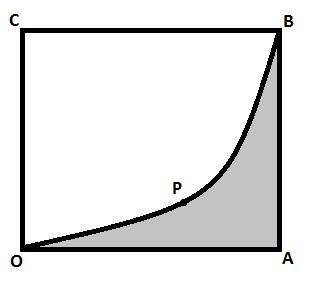Solution:

Area of shaded region = Area of square OABC – Area of quadrant COPB having radius OC

= (Side)2$\frac{1}{4}$ ($\Pi \times r^{2}$)

= (7)2$\frac{1}{4}$ $\left [ \frac{22}{7}\times 7^{2} \right ]$ = 49 – 38.5 = 10.5 cm2

Hence, the area of the shaded region is 10.5 cm2

Question 7: In the given figure, three sectors of a circle of radius 7 cm, making angles of 60o, 80o and 40o at the centre are shaded. Find the area of the shaded region.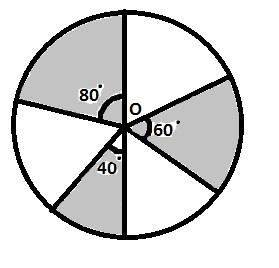Solution:

Area of the shaded region = Area of sector having central angle 60o + Area of sector having central angle 80o + Area of sector having central angle 40o

= $\left [ \frac{60^{\circ}}{360^{\circ}}\times \Pi (7)^{2} \right ]+\left [ \frac{80^{\circ}}{360^{\circ}}\times \Pi (7)^{2} \right ]+\left [ \frac{40^{\circ}}{360^{\circ}}\times \Pi (7)^{2} \right ]$

= $\Pi (7)^{2}\left ( \frac{60^{\circ}}{360^{\circ}}+\frac{80^{\circ}}{360^{\circ}}+\frac{40^{\circ}}{360^{\circ}} \right )$

= $\Pi (7)^{2}\left ( \frac{180^{\circ}}{360^{\circ}} \right )$ = $\frac{22}{7 }(7)^{2}\left ( \frac{1}{2} \right )$ = 77 cm2

Hence, the area of the shaded region is 77 cm2.

Question 8: In the given figure, PQ and AB are respectively the arcs of two concentric circles of radii 7 cm and 3.5 cm with centre O. If $\angle$POQ = 30o, find the area of the shaded region.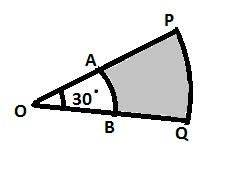Solution:

Area of the shaded portion = Area of sector OPQ – Area of sector OAB

= $\frac{30^{\circ}}{360^{\circ}}\times \Pi (7)^{2}-\frac{30^{\circ}}{360^{\circ}}\times \Pi (3.5)^{2}$

= $\frac{22}{7}\times \frac{1}{12}\left [ (7)^{2}-(3.5)^{2} \right ]$

= $\frac{22}{7}\times \frac{1}{12}\left [ (7)^{2}-\left ( \frac{7}{2} \right )^{2} \right ]$

= $\frac{22}{7}\times \frac{1}{12}\times \frac{147}{4}$ = $\frac{77}{8}$ cm2

Hence, the area of the shaded portion is $\frac{77}{8}$ cm2.

Question 9: In the given figure, find the area of the shaded region, if ABCD is a square of side 14 cm and APD and BPC are semi-circles.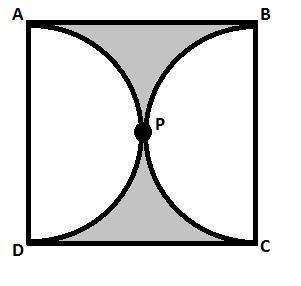Solution:

Area of the shaded region = Area of Square ABCD – (Area of semicircle APD + Area of semicircle BPC)

= (14)2$\left [ \frac{1}{2}\Pi \left ( \frac{14}{2} \right )^{2}+\frac{1}{2}\Pi \left ( \frac{14}{2} \right )^{2} \right ]$ = (14)2$\frac{22}{7}(7)^{2}$ = 42 cm2

Hence, the area of the shaded region is 42 cm2.

Question 10: In the given figure, the shape of the top of a table is that of a sector of a circle with centre O and $\angle$AOB = 90o. If AO = OB = 42 cm, then find the perimeter of the top of the table.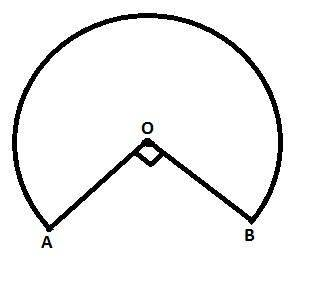Solution:

We have r = 42 cm and $\Theta$ = 360o – 90o = 270o

Perimeter of the top of the table = Length of the major arc AB + Length of OA + Length of OB

= $\frac{\Theta }{360^{\circ}}\times 2\Pi r+42+42$

= $\frac{270^{\circ}}{360^{\circ}}\times 2\times \frac{22}{7}\times 42+84$ = 198 + 84 = 282 cm

Hence, the perimeter of the top of the table is 282 cm.

Question 11: In the given figure, ABCD is a square of side 7 cm, DPBA and DQBC are quadrants of circles each of the radius 7 cm. find the area of the shaded region.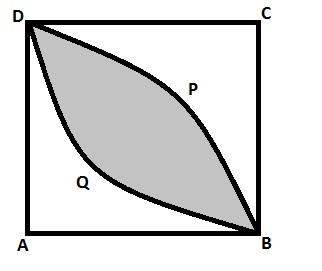Solution:

Area of the shaded portion = (Area of quadrant DPBA + Area of quadrant DQBC) – Area of Square ABCD

= $\left [ \frac{1}{4}\Pi (7)^{2}+\frac{1}{4}\Pi (7)^{2} \right ]-(7)^2$ = $\frac{1}{2}\times \frac{22}{7}(7)^2-49$ = 28 cm2

Hence, the area of the shaded portion is 28 cm2.

Question 12: In the given figure, OABC is a quadrant of a circle with centre O and radius 3.5 cm. If OD = 2 cm, find the area of the shaded region.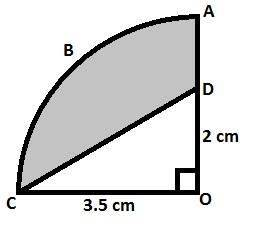Solution:

Area of the right-angled $\Delta$COD = $\frac{1}{2}$ x b x h = $\frac{1}{2}$ x 3.5 x 2 = 3.5 cm2

Area of the sector AOC = $\frac{\Theta }{360}$ x $\Pi$ x r2

= $\frac{90}{360}$ x $\frac{22}{7}$ x (3.5)2 = 9.625 cm2

Area of the shaded region = Area of the $\Delta$COD – Area of the sector AOC = 9.625 – 3.50 = 6.125 cm2

Question 13: Find the perimeter of the shaded region in the figure, if ABCD is a square of side 14 cm and APB and CPD are semicircles.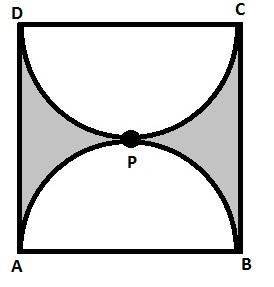Solution:

Perimeter of shaded region = Length of the arc APB + Length of the arc CPD + Length of AD + Length of BC

= $\frac{1}{2}\times 2\Pi r+\frac{1}{2}\times 2\Pi r+14+14$

= $2\Pi r$ + 28 = $2\times \frac{22}{7}\times \frac{14}{2}+28$ = 72 cm

Hence, the perimeter of the shaded region is 72 cm.

Question 14: In a circle of radius 7 cm, a square ABCD is inscribed. Find the areas of the circle which is outside the square.

Solution:

Let the diagonal of the square be d.

We know that if a circle circumscribes a square, then the diameter of the circle is equal to the diagonal of the square.

Therefore, d = 2 x 7 = 14 cm

Now,

Area of required region = Area of circle – Area of square

= $\Pi r^{2}-\frac{1}{2}d^{2}$ = $\frac{22}{7}\times (7)^2-\frac{1}{2}\times (14)^2$ = 56 cm2

Hence, the required area is 56 cm2.

Question 15: In the given figure, APB and CQD are semicircles of diameter 7 cm each, while ARC BSD are semicircles of diameter 14 cm each. Find the (i) perimeter, (ii) area of the shaded region.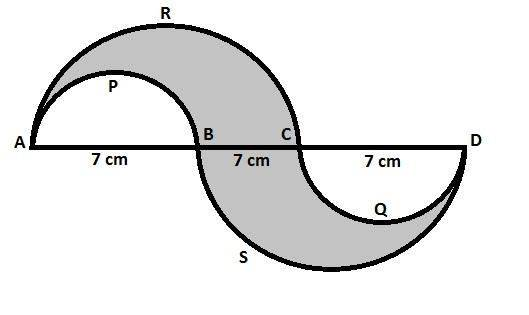Solution:

(i) Perimeter of shaded region = Length of the arc APB + Length of the arc ARC + Length of the arc BSD + Length of the arc CQD

= $\frac{1}{2}\times 2\Pi r_{1}+\frac{1}{2}\times 2\Pi r_{2}+\frac{1}{2}\times 2\Pi r_{3}+\frac{1}{2}\times 2\Pi r_{4}$

= $\frac{1}{2}\times 2\Pi \left ( \frac{7}{2} \right )+\frac{1}{2}\times 2\Pi (7)+\frac{1}{2}\times 2\Pi (7)+\frac{1}{2}\times 2\Pi \left ( \frac{7}{2} \right )$

= $2\Pi \left ( \frac{7}{2} \right )+2\Pi (7)$

= $2\Pi \left ( \frac{7}{2}+7 \right )$ = $2\times \frac{22}{7}\times \frac{21}{2}$ = 66 cm

(ii) Area of shaded region = Area of the arc ARC + Area of the arc BSD – (Area of the arc APB + Area of the arc CQD)

= $\frac{1}{2}\times \Pi (r_{1})^{2}+\frac{1}{2}\times \Pi (r_{2})^{2}-\left [ \frac{1}{2}\times \Pi (r_{3})^{2}+\frac{1}{2}\times \Pi (r_{4})^{2} \right ]$

= $\frac{1}{2}\times \Pi (7)^{2}+\frac{1}{2}\times \Pi (7)^{2}-\left [ \frac{1}{2}\times \Pi \left ( \frac{7}{2} \right )^{2}+\frac{1}{2}\times \Pi \left ( \frac{7}{2} \right )^{2} \right ]$

= $\Pi (7)^{2}-\Pi \left ( \frac{7}{2} \right )^{2}$

= $\Pi \left ( 49-\frac{49}{4} \right )$ = $\frac{22}{7}\times \frac{147}{4}$ = 115.5 cm2

Question 16: In the given figure, PSR, RTQ and PAQ are three semicircles of diameter 10 cm, 3 cm and 7 cm respectively. Find the perimeter of the shaded region. [Use $\Pi$ = 3.14]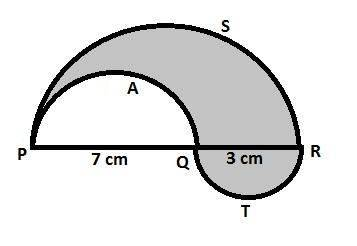Solution:

Perimeter of shaded region = Length of the arc PAQ + Length of the arc PSR + Length of the arc RTQ

= $\frac{1}{2}\times 2\Pi r_{1}+\frac{1}{2}\times 2\Pi r_{2}+\frac{1}{2}\times 2\Pi r_{3}$

= $\frac{1}{2}\times 2\Pi \left ( \frac{7}{2} \right )+\frac{1}{2}\times 2\Pi \left ( \frac{10}{2} \right )+\frac{1}{2}\times 2\Pi \left ( \frac{3}{2} \right )$ = $\frac{7}{2}\Pi +5\Pi +\frac{3}{2}\Pi$

= $\frac{7}{2}\Pi +\frac{3}{2}\Pi +5\Pi$ = $5\Pi +5\Pi$ = $10\Pi$ = 31.4 cm

Hence, the perimeter of shaded region is 31.4 cm.

Question 17: In the given figure, a square OABC is inscribed in a quadrant OPBQ of a circle. If OA = 20 cm, find the area of the shaded region. [Use $\Pi$ = 3.14]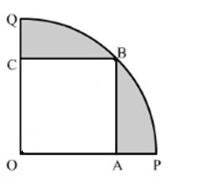Solution:

Construction: Join OB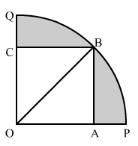In right triangle AOB

OB2 = OA2 + AB2 = 202 + 202 = 400 + 400 = 800

Therefore, OB2 = 800

Area of the shaded region = Area of quadrant OPBQ – Area of Square OABC

= $\frac{1}{4}\Pi (OB)^2-(OA)^2$

= $\frac{1}{4}\times 3.14\times 800-400$ = 628 – 400 = 228 cm2

Hence, the area of the shaded region is 228 cm2.

Practise This Question

Heating ethanol with excess concentrated sulphuric acid will give